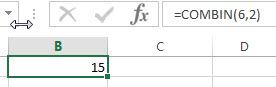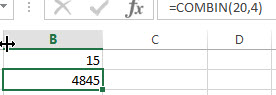## Excel COMBIN Function

This post will guide you how to use Excel COMBIN function with syntax and examples in Microsoft excel.

### Description

The Excel COMBIN function returns the number of combinations for a given number of items. And you can use the COMBIN function to determine the total possible number of groups for a given number of items.

For example, assuming that you have 6 objects(a,b,c,d,e,f) and you can get 15 different combinations of 2 objects , the combinations are as follows:

`ab ac ad ae af bc bd be bf cd ce cf de df ef `

The COMBIN function is a build-in function in Microsoft Excel and it is categorized as a Math and Trigonometry Function.

The COMBIN function in Excel 2016, Excel 2013, Excel 2010, Excel 2007, Excel 2003, Excel XP, Excel 2000, Excel 2011 for Mac.

### Syntax

The syntax of the COMBIN function is as below:

`=COMBIN (number, number_chosen)`

Where the COMBIN function arguments are:

• number – This is a required argument. . The number of items. It must be greater than 0, and it also must be greater than number_chosen argument.
• number_chosen – This is a required argument. The number of combinations that you want to.

Note:

• If either the number argument or the number_chosen argument is less than 0, then the COMBINA function will return the #NUM! Error.
• If any arguments are non-numeric, this function will return #VALUE! Error.
• If any arguments are integer values and will be truncated.
• if you want to calculate the number of combinations for a given number of items (with repetitions), you can use the COMBINA function.

### Excel COMBIN Function Examples

The below examples will show you how to use Excel COMBIN Function to get the number of combinations for a given number objects from a set (in any order).

1# to get the number of combinations for 6 and 2(in any order), enter the following formula in Cell B1.

`=COMBIN(6,2)`2# to get the number of combinations for 20 and 4(in any order), enter the following formula in Cell B2.

`=COMBIN(20,4)`### Related Functions

• Excel COMBINA Function
The Excel COMBINA function returns the number of combinations for a given number of items and it includes repetitions. The syntax of the COMBINA function is as below:=COMBINA(number, number_chosen)…